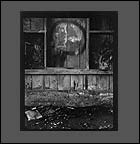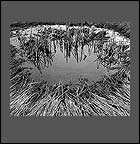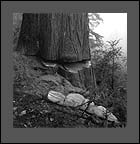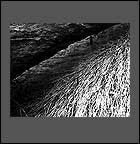P R O F I L EG A L L E R YO R D E RC O N T A C T    I N F OS  T  I  L  L     L  I  F  E

"Can you feel the sharp edge of the rushes? The cool dampness of the dew? The slickness of the stone? It is of this energy that I seek."

G A L L E R Y
F E A T U R E D   E X H I B I T
L A N D S C A P E
P E O P L E   &   L I F E S T Y L E
H U M A N   S H A P E   &   F O R M
> S T I L L   L I F E
E X P E R I M E N T A L   W O R Kf l o o dt h e   s m o k e rr u s h e st h e   w e d g et h i s   w a yr e e d   d i r e c t i o n s >  S E N D   T H I S   S I T E   T O   A   F R I E N DT O P© 2001 - 2005   R o b e r  t   J   W i l k i n s o n             P r i v a c y   P o l i c ys i t e   d e s i g n